Test: 1P Diode Rectifiers HW

# Test: 1P Diode Rectifiers HW

Test Description

## 20 Questions MCQ Test Power Electronics | Test: 1P Diode Rectifiers HW

Test: 1P Diode Rectifiers HW for Electrical Engineering (EE) 2023 is part of Power Electronics preparation. The Test: 1P Diode Rectifiers HW questions and answers have been prepared according to the Electrical Engineering (EE) exam syllabus.The Test: 1P Diode Rectifiers HW MCQs are made for Electrical Engineering (EE) 2023 Exam. Find important definitions, questions, notes, meanings, examples, exercises, MCQs and online tests for Test: 1P Diode Rectifiers HW below.
Solutions of Test: 1P Diode Rectifiers HW questions in English are available as part of our Power Electronics for Electrical Engineering (EE) & Test: 1P Diode Rectifiers HW solutions in Hindi for Power Electronics course. Download more important topics, notes, lectures and mock test series for Electrical Engineering (EE) Exam by signing up for free. Attempt Test: 1P Diode Rectifiers HW | 20 questions in 20 minutes | Mock test for Electrical Engineering (EE) preparation | Free important questions MCQ to study Power Electronics for Electrical Engineering (EE) Exam | Download free PDF with solutions
 1 Crore+ students have signed up on EduRev. Have you?
Test: 1P Diode Rectifiers HW - Question 1

### In the process of diode based rectification, the alternating input voltage is converted into

Detailed Solution for Test: 1P Diode Rectifiers HW - Question 1

Rectification is AC to DC. In DIODE biased rectification, control is not possible.

Test: 1P Diode Rectifiers HW - Question 2

### In a half-wave rectifier, the

Detailed Solution for Test: 1P Diode Rectifiers HW - Question 2

Current is always in one direction only, but voltage can be bi-directional in case of an L load.

Test: 1P Diode Rectifiers HW - Question 3

### For a certain diode based rectifier, the output voltage (average value) is given by the equation 1/2π [ ∫Vm sin ωt d(ωt) ] Where the integral runs from 0 to π

Detailed Solution for Test: 1P Diode Rectifiers HW - Question 3

Integration is 0 to π from base period of 1/2π so it is a half wave R load.

Test: 1P Diode Rectifiers HW - Question 4

For a single phase half wave rectifier, with R load, the diode is reversed biased from ωt =

Detailed Solution for Test: 1P Diode Rectifiers HW - Question 4

Diode will be reversed biased in the negative half cycles.

Test: 1P Diode Rectifiers HW - Question 5

For the circuit shown below,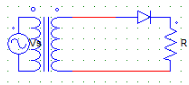The secondary transformer voltage Vs is given by the expression
Vs = Vm sin ωt
Find the PIV of the diode.

Detailed Solution for Test: 1P Diode Rectifiers HW - Question 5

PIV = √2 Vs = Vm.

Test: 1P Diode Rectifiers HW - Question 6

For the circuit shown below,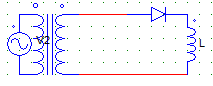The peak value of the load current occurs at ωt = ?

Detailed Solution for Test: 1P Diode Rectifiers HW - Question 6

Due to the L nature, load current is maximum when the diode will be com-mutated i.e at π.

Test: 1P Diode Rectifiers HW - Question 7

Find the rms value of the output voltage for the circuit shown below.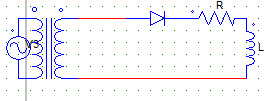Voltage across the secondary is given by Vm sinωt.

Detailed Solution for Test: 1P Diode Rectifiers HW - Question 7

The above is a HW diode rectifier, the RMS o/p voltage equation is given by
Vor = √ [ (1/2π) ∫π Vm2sin2ωt. d(ωt) ] Solving above equation we get, Vor = Vm/2.

Test: 1P Diode Rectifiers HW - Question 8

In a 1-Phase HW diode rectifier with R load, the average value of load current is given byTake Input (Vs) = Vm sinωt

Detailed Solution for Test: 1P Diode Rectifiers HW - Question 8

Vo = √ [(1/2π) ∫π Vm sinωt. d(ωt)] Vo = Vm/π
I = Vo/R = Vm/πR.

Test: 1P Diode Rectifiers HW - Question 9

In the circuit shown below,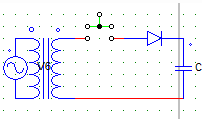The switch (shown in green) is closed at ωt = 0. The load current or capacitor current has the maximum value at ωt =

Detailed Solution for Test: 1P Diode Rectifiers HW - Question 9

The instant switch is closed the load current will be zero due to the nature of the capacitor.

Test: 1P Diode Rectifiers HW - Question 10

Find the average value of output current for a 1-phase HW diode rectifier with R load, having RMS output current = 100A.

Detailed Solution for Test: 1P Diode Rectifiers HW - Question 10

I(rms) = Vm/2R
Therfore, Vm = 200R
I(avg) = Vm/πR = 200R/πR.

Test: 1P Diode Rectifiers HW - Question 11

A 1-phase 230V, 1KW heater is connected across a 1-phase HW rectifier (diode based). The power delivered to the heater is

Detailed Solution for Test: 1P Diode Rectifiers HW - Question 11

R = (230 x 230)/1000
V(rms) = (√2 x 230)/2
P = V(rms)2/R = 500W.

Test: 1P Diode Rectifiers HW - Question 12

A 1-phase half wave diode rectifier with R load, has input voltage of 240 V. The input power factor is

Detailed Solution for Test: 1P Diode Rectifiers HW - Question 12

Input p.f = V(rms)/Vs
Vrms is the RMS value of output voltage. Vrms = (√2 x 230)/2
Vs = 230
pf = 0.707.

Test: 1P Diode Rectifiers HW - Question 13

A 1-phase half wave diode rectifier with R = 1 KΩ, has input voltage of 240 V. The diode peak current is

Detailed Solution for Test: 1P Diode Rectifiers HW - Question 13

Diode peak current = peak current through the load = Vo/R = Vm/2R.

Test: 1P Diode Rectifiers HW - Question 14

For the below given circuit, after the switch is closed the voltage across the load (shown open) remains constant.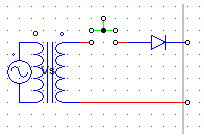Assuming that all initial conditions are zero. The element across the load would be a/an

Detailed Solution for Test: 1P Diode Rectifiers HW - Question 14

As the voltage remains constant as soon as the switch is closed, the element is most likely to be a capacitor.

Test: 1P Diode Rectifiers HW - Question 15

For the below given circuit,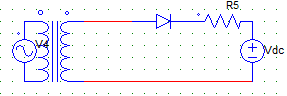After the supply voltage (Vs) is given the

Detailed Solution for Test: 1P Diode Rectifiers HW - Question 15

The diode will be forward biased only when Vs will be greater than Vdc.

Test: 1P Diode Rectifiers HW - Question 16

For the below given circuit,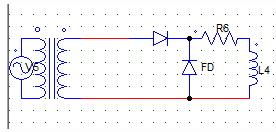With Vs = Vm sin ωt (secondary side). The expression for the average voltage is

Detailed Solution for Test: 1P Diode Rectifiers HW - Question 16

Due to the FD, we get 1st quadrant operation.
Where, output voltage (Avg) = 1/2π [ ∫Vm sin ωt d(ωt) ], integration runs from 0 to 180 degrees.

Test: 1P Diode Rectifiers HW - Question 17

For the below given circuit, the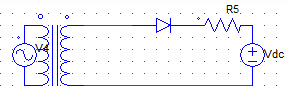Detailed Solution for Test: 1P Diode Rectifiers HW - Question 17

The output voltage is the voltage across the resister and Vdc. Even if the current falls to zero, the output voltage will be equal to Vdc.

Test: 1P Diode Rectifiers HW - Question 18

For the below given circuit,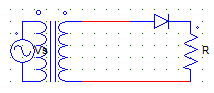Vs = 325 sin ωt (secondary side)
The ripple voltage is

Detailed Solution for Test: 1P Diode Rectifiers HW - Question 18

Ripple voltage = √(Vrms2 + Vavg2)
Vrms = Vm/2
Vavg = Vm/π

Test: 1P Diode Rectifiers HW - Question 19

For a single phase half wave rectifier, the rectifier efficiency is always constant & it is

Detailed Solution for Test: 1P Diode Rectifiers HW - Question 19

Rectifier efficiency = Pdc/Pac
Pdc = (Vm x Im)/π2
Pac = 4/(Vm x Im).

Test: 1P Diode Rectifiers HW - Question 20

For the below given circuit,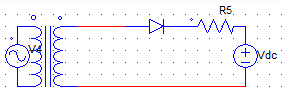The power delivered to the load in Watts is

Detailed Solution for Test: 1P Diode Rectifiers HW - Question 20

P = power delivered to the resister + power delivered to the emf source.

## Power Electronics

5 videos|39 docs|63 tests
 Use Code STAYHOME200 and get INR 200 additional OFF Use Coupon Code
Information about Test: 1P Diode Rectifiers HW Page
In this test you can find the Exam questions for Test: 1P Diode Rectifiers HW solved & explained in the simplest way possible. Besides giving Questions and answers for Test: 1P Diode Rectifiers HW, EduRev gives you an ample number of Online tests for practice

## Power Electronics

5 videos|39 docs|63 tests